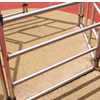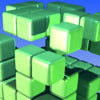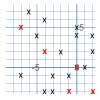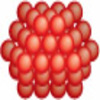# Resources tagged with: Visualising

Filter by: Content type:
Age range:
Challenge level:

### There are 178 results

Broad Topics > Mathematical Thinking > Visualising### LOGO Challenge - Circles as Animals

##### Age 11 to 16 Challenge Level:

See if you can anticipate successive 'generations' of the two animals shown here.### Polygon Pictures

##### Age 11 to 14 Challenge Level:

Can you work out how these polygon pictures were drawn, and use that to figure out their angles?### Tied Up

##### Age 14 to 16 Short Challenge Level:

How much of the field can the animals graze?### Star Gazing

##### Age 14 to 16 Challenge Level:

Find the ratio of the outer shaded area to the inner area for a six pointed star and an eight pointed star.### Efficient Packing

##### Age 14 to 16 Challenge Level:

How efficiently can you pack together disks?### Trice

##### Age 11 to 14 Challenge Level:

ABCDEFGH is a 3 by 3 by 3 cube. Point P is 1/3 along AB (that is AP : PB = 1 : 2), point Q is 1/3 along GH and point R is 1/3 along ED. What is the area of the triangle PQR?### Cube Paths

##### Age 11 to 14 Challenge Level:

Given a 2 by 2 by 2 skeletal cube with one route `down' the cube. How many routes are there from A to B?### Weighty Problem

##### Age 11 to 14 Challenge Level:

The diagram shows a very heavy kitchen cabinet. It cannot be lifted but it can be pivoted around a corner. The task is to move it, without sliding, in a series of turns about the corners so that it. . . .### Rolling Around

##### Age 11 to 14 Challenge Level:

A circle rolls around the outside edge of a square so that its circumference always touches the edge of the square. Can you describe the locus of the centre of the circle?### Tessellating Hexagons

##### Age 11 to 14 Challenge Level:

Which hexagons tessellate?### Polygon Rings

##### Age 11 to 14 Challenge Level:

Join pentagons together edge to edge. Will they form a ring?### An Unusual Shape

##### Age 11 to 14 Challenge Level:

Can you maximise the area available to a grazing goat?### Like a Circle in a Spiral

##### Age 7 to 16 Challenge Level:

A cheap and simple toy with lots of mathematics. Can you interpret the images that are produced? Can you predict the pattern that will be produced using different wheels?### The Old Goats

##### Age 11 to 14 Challenge Level:

A rectangular field has two posts with a ring on top of each post. There are two quarrelsome goats and plenty of ropes which you can tie to their collars. How can you secure them so they can't. . . .### Natural Sum

##### Age 14 to 16 Challenge Level:

The picture illustrates the sum 1 + 2 + 3 + 4 = (4 x 5)/2. Prove the general formula for the sum of the first n natural numbers and the formula for the sum of the cubes of the first n natural. . . .### Concrete Wheel

##### Age 11 to 14 Challenge Level:

A huge wheel is rolling past your window. What do you see?### Picture Story

##### Age 14 to 16 Challenge Level:

Can you see how this picture illustrates the formula for the sum of the first six cube numbers?### All Tied Up

##### Age 14 to 16 Challenge Level:

A ribbon runs around a box so that it makes a complete loop with two parallel pieces of ribbon on the top. How long will the ribbon be?##### Age 11 to 14 Challenge Level:

Four rods, two of length a and two of length b, are linked to form a kite. The linkage is moveable so that the angles change. What is the maximum area of the kite?### On the Edge

##### Age 11 to 14 Challenge Level:

If you move the tiles around, can you make squares with different coloured edges?### Framed

##### Age 11 to 14 Challenge Level:

Seven small rectangular pictures have one inch wide frames. The frames are removed and the pictures are fitted together like a jigsaw to make a rectangle of length 12 inches. Find the dimensions of. . . .### Tetra Square

##### Age 11 to 14 Challenge Level:

ABCD is a regular tetrahedron and the points P, Q, R and S are the midpoints of the edges AB, BD, CD and CA. Prove that PQRS is a square.### A Tilted Square

##### Age 14 to 16 Challenge Level:

The opposite vertices of a square have coordinates (a,b) and (c,d). What are the coordinates of the other vertices?### Corridors

##### Age 14 to 16 Challenge Level:

A 10x10x10 cube is made from 27 2x2 cubes with corridors between them. Find the shortest route from one corner to the opposite corner.### Zooming in on the Squares

##### Age 7 to 14

Start with a large square, join the midpoints of its sides, you'll see four right angled triangles. Remove these triangles, a second square is left. Repeat the operation. What happens?### Playground Snapshot

##### Age 7 to 14 Challenge Level:

The image in this problem is part of a piece of equipment found in the playground of a school. How would you describe it to someone over the phone?### Coloured Edges

##### Age 11 to 14 Challenge Level:

The whole set of tiles is used to make a square. This has a green and blue border. There are no green or blue tiles anywhere in the square except on this border. How many tiles are there in the set?### Cutting a Cube

##### Age 11 to 14 Challenge Level:

A half-cube is cut into two pieces by a plane through the long diagonal and at right angles to it. Can you draw a net of these pieces? Are they identical?### Convex Polygons

##### Age 11 to 14 Challenge Level:

Show that among the interior angles of a convex polygon there cannot be more than three acute angles.### The Perforated Cube

##### Age 14 to 16 Challenge Level:

A cube is made from smaller cubes, 5 by 5 by 5, then some of those cubes are removed. Can you make the specified shapes, and what is the most and least number of cubes required ?### Tilting Triangles

##### Age 14 to 16 Challenge Level:

A right-angled isosceles triangle is rotated about the centre point of a square. What can you say about the area of the part of the square covered by the triangle as it rotates?### LOGO Challenge - Triangles-squares-stars

##### Age 11 to 16 Challenge Level:

Can you recreate these designs? What are the basic units? What movement is required between each unit? Some elegant use of procedures will help - variables not essential.### Dissect

##### Age 11 to 14 Challenge Level:

What is the minimum number of squares a 13 by 13 square can be dissected into?### Eight Hidden Squares

##### Age 7 to 14 Challenge Level:

On the graph there are 28 marked points. These points all mark the vertices (corners) of eight hidden squares. Can you find the eight hidden squares?### Baravelle

##### Age 7 to 16 Challenge Level:

What can you see? What do you notice? What questions can you ask?### Efficient Cutting

##### Age 11 to 14 Challenge Level:

Use a single sheet of A4 paper and make a cylinder having the greatest possible volume. The cylinder must be closed off by a circle at each end.### Ten Hidden Squares

##### Age 7 to 14 Challenge Level:

These points all mark the vertices (corners) of ten hidden squares. Can you find the 10 hidden squares?### Getting an Angle

##### Age 11 to 14 Challenge Level:

How can you make an angle of 60 degrees by folding a sheet of paper twice?### Something in Common

##### Age 14 to 16 Challenge Level:

A square of area 3 square units cannot be drawn on a 2D grid so that each of its vertices have integer coordinates, but can it be drawn on a 3D grid? Investigate squares that can be drawn.### Packing 3D Shapes

##### Age 14 to 16 Challenge Level:

What 3D shapes occur in nature. How efficiently can you pack these shapes together?### Just Opposite

##### Age 14 to 16 Challenge Level:

A and C are the opposite vertices of a square ABCD, and have coordinates (a,b) and (c,d), respectively. What are the coordinates of the vertices B and D? What is the area of the square?### Triangular Tantaliser

##### Age 11 to 14 Challenge Level:

Draw all the possible distinct triangles on a 4 x 4 dotty grid. Convince me that you have all possible triangles.### Square It

##### Age 11 to 16 Challenge Level:

Players take it in turns to choose a dot on the grid. The winner is the first to have four dots that can be joined to form a square.### Squares, Squares and More Squares

##### Age 11 to 14 Challenge Level:

Can you dissect a square into: 4, 7, 10, 13... other squares? 6, 9, 12, 15... other squares? 8, 11, 14... other squares?### Dotty Triangles

##### Age 11 to 14 Challenge Level:

Imagine an infinitely large sheet of square dotty paper on which you can draw triangles of any size you wish (providing each vertex is on a dot). What areas is it/is it not possible to draw?### Hidden Rectangles

##### Age 11 to 14 Challenge Level:

Rectangles are considered different if they vary in size or have different locations. How many different rectangles can be drawn on a chessboard?### Auditorium Steps

##### Age 7 to 14 Challenge Level:

What is the shape of wrapping paper that you would need to completely wrap this model?### 3D Stacks

##### Age 7 to 14 Challenge Level:

Can you find a way of representing these arrangements of balls?### Steel Cables

##### Age 14 to 16 Challenge Level:

Some students have been working out the number of strands needed for different sizes of cable. Can you make sense of their solutions?### Around and Back

##### Age 14 to 16 Challenge Level:

A cyclist and a runner start off simultaneously around a race track each going at a constant speed. The cyclist goes all the way around and then catches up with the runner. He then instantly turns. . . .